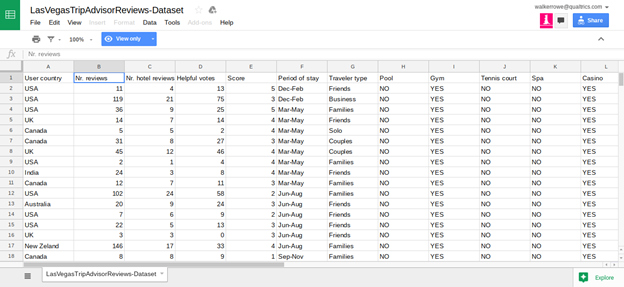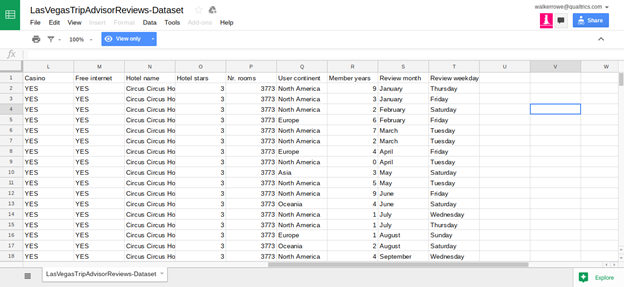﻿ Using Tensorflow to Create Neural Network with Tripadvisor Data: Part I – BMC Blogs
Machine Learning & Big Data Blog

# Using Tensorflow to Create Neural Network with Tripadvisor Data: Part IbyWhen people are trying to learn neural networks with TensorFlow they usually start with the handwriting database. This builds a model that predicts what digit a person has drawn based upon handwriting samples obtained from thousands of persons. To put that into features-labels terms, the combinations of pixels in a grayscale image (white, black, grey) determine what digit is drawn (0, 1, .., 8, 9).

Here we use other data.

## Prerequisites

Before reading this TensorFlow Neural Network tutorial, you should first study these three blog posts:

Then you need to install TensorFlow. The easiest way to do that on Ubuntu is to follow these instructions and use virtualenv.

Then install Python Pandas, numpy, scikit-learn, and SciPy packages.

## The Las Vegas Strip Hotel Dataset from Trip Advisor

Programmers who are learning to using TensorFlow often start with the iris-data database. That given the combination of pixels that show what type of Iris flower is drawn. But we want to do something original here instead of use the Iris dataset. So we will use the Las Vegas Strip Data Set, cited in the paper “Moro, S., Rita, P., & Coelho, J. (2017). Stripping customers’ feedback on hotels through data mining: The case of Las Vegas Strip. Tourism Management Perspectives, 23, 41-52.” and see if we can wrap a neural network around it.

In their paper, the authors wrote a model using the R programming language and used Support Vector Matrices (SVMs) as their algorithm. That is a type of non-linear regression problem. It uses the same approach to solving regular LR problems, which is to find a line that reduces the MSE (mean square error) to its lowest point to build a predictive model. But SVMs take that up a notch in complexity by working with multiple, nonlinear inputs and finds a plane in n-dimensional space and not line on the XY Cartesian Plane.

Here we take the same data and but use a neural network instead of SVM. We will present this in 3 blog posts:

1. Put data into numeric format.
2. Train neural network.
3. Make prediction.

The data and code for this tutorial is located here.

## The Data

Click here to see the data in Google Sheets format. The data is too wide to fit on one screen so we show it below in two screen prints. If you read the paper cited above you can get more details about the data but basically it is TripAdvisor data for 21 Hotels along the Las Vegas Strip. The goal is to build a model that will predict what score an individual is likely to give to which hotel.

The score is 1 to 5 and the input are 20 variables described in the spreadsheet below.The authors of the paper say that certain data—like whether the hotel has a casio, pool, number of stars, or free internet—does not have much bearing on the score given by the hotel guest on Tripadvisor. Rather the factors that most heavily predict the score are the number of reviews the reviewer has written and how long they have been writing reviews. Other factors that influence the score are the day of the week and the month of the year.

## Convert Values to Integers

You can download the code below from this iPython notebook.

First we need to convert all of those values to integers as machine learning uses arrays of numbers as input. We adopt three approaches:

1. If the number is already an integer leave it.
2. If the number is a YES or NO then change it to 1 or 0.
3. If the element in a string, then use the ordinal string function to change each letter to a integer. Then sum those integers.

```import pandas as pd def yesNo(x): if x=="YES": return 1 else: return 0 def toOrd(str): x=0 for l in str: x += ord(l) return int(x) cols = ['User country', 'Nr. reviews','Nr. hotel reviews','Helpful votes', 'Score','Period of stay','Traveler type','Pool','Gym','Tennis court', 'Spa','Casino','Free internet','Hotel name','Hotel stars','Nr. rooms', 'User continent','Member years','Review month','Review weekday'] df = pd.read_csv('/home/walker/TripAdvisor.csv',sep=',',header=0) df['Casino']=df['Casino'].apply(lambda x : yesNo(x)) df['Gym']=df['Gym'].apply(lambda x : yesNo(x)) df['Pool']=df['Pool'].apply(lambda x : yesNo(x)) df['Tennis court']=df['Tennis court'].apply(lambda x : yesNo(x)) df['Casino']=df['Casino'].apply(lambda x : yesNo(x)) df['Free internet']=df['Free internet'].apply(lambda x : yesNo(x)) df['Spa']=df['Spa'].apply(lambda x : yesNo(x)) cols2 = ['Period of stay', 'Hotel name', 'User country', 'Traveler type', 'User continent', 'Review month', 'Review weekday'] for y in cols2: df[y]=df[y].apply(lambda x: toOrd(x)) df.to_csv('tripAdvisorFL.csv')```

Here we change every string to an integer. You would have to save the string-integer combination in some data structure so that later you could see which integer equals what string value.

### Wikibon: Automate your Big Data pipeline

Learn how data management experts throughout the industry are transforming their Big Data infrastructure for maximum business impact.

These postings are my own and do not necessarily represent BMC's position, strategies, or opinion.

See an error or have a suggestion? Please let us know by emailing blogs@bmc.com.

### About the author#### Walker Rowe

Walker Rowe is an American freelance tech writer and programmer living in Tunisia. He specializes in big data, analytics, and programming languages. Find him on LinkedIn or Upwork.